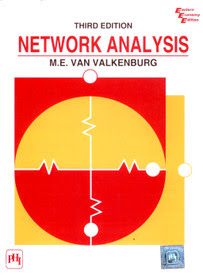Network Analysis By Van Valkenburg : GATE BooksNetwork Analysis

(Paperback)
by

M. E. Van Valkenburg

10 Ratings  |
Publisher: PHI Learning (2011)

Book Summary of Network Analysis

A treatment of topics in the field of electric networks (or circuits) which are considered as a foundation in electrical engineering and occur at either the beginning or intermediate level. Contains material on the Nyquist criterion. Gives Tellegan's theorem, and the Gauss elimination method. Includes expanded coverage of Thevenin's and Norton's theorems, the Routh-Hurwitz criterion, and Fourier transforms. Problems and suggested digital computer exercises at the end of each chapter. Solutions to selected problems given in the appendix.

• Development of the Circuit Concept
• Conventions for Describing Networks
• Network Equations
• First-order Differential Equations
• Initial Conditions in Networks
• The Laplace Transformation
• Transforms of Other Signal Wave-forms
• Impedance Functions and Network Theorems
• Network Functions; Poles and Zeros
• Two-Port Parameters
• Frequency Response Plots
• Input Power, Power Transfer, and Insertion Loss
• Fourier Series and Signal Spectra
• Fourier Integral and Continuous Spectra
• Appendices

Details of Book: Network Analysis

Book:Network Analysis
Author:M. E. Van Valkenburg
ISBN:

ISBN-13:

978-8120301566

Binding:Paperback
Publishing Date:2011
Publisher:PHI Learning
Edition:3rdEdition
Number of Pages:592
Language:English

Also Visit For
~~~~~~~~~~~~~~~~~~~~~